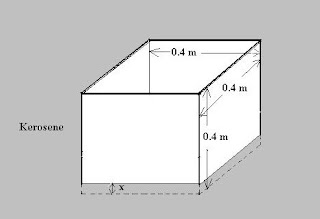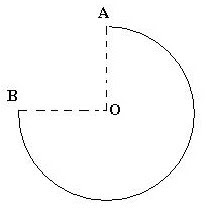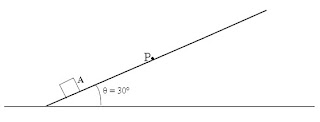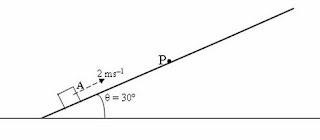## Pages

`“Life is like riding a bicycle.  To keep your balance you must keep moving.”–Albert Einstein`

## Friday, September 30, 2011

### AP Physics B - Free Response Practice Question on Fluid Mechanics

“Our greatest weakness lies in giving up. The most certain way to succeed is always to try just one more time.”

– Thomas A. Edison

Today I will give you a free response practice question on fluid mechanics. The question is meant for AP Physics B aspirants. But it will be useful for AP Physics C aspirants also as it high lights basic principles in hydrostatics. Generally questions meant for AP Physics C will be tougher than those for AP Physics B. So the question I give below can serve only as a part of a more difficult question in their case. Here is the question:The adjoining figure shows an empty thin walled cubical vessel of side 0.4 m and mass 6.4 kg floating on kerosene contained in a large tank (not shown). Density of kerosene is 800 kgm–3 where as the density of water is 1000 kgm–3. You may take the acceleration due to gravity as 10 ms–2. Now, answer the following questions:

(a) Calculate the magnitudes of the force of buoyancy and the force of gravity acting on the empty vessel and state their directions.

(b) Calculate the height x of the portion of the empty vessel that is submerged in kerosene.

(c) Water is slowly poured into the vessel so that an additional 0.2 m of the height of the vessel is submerged in kerosene. Calculate the volume of water added to obtain this condition.

Try to answer the above question which carries 10 points. You have about 11 minutes for answering it. I’ll be back shortly with a model answer for your benefit.

You will find a useful post in this section here.

## Monday, September 19, 2011

### AP Physics B & C – Practice Questions (MCQ) Involving Kinematics and Elastic Collision

“Men often become what they believe themselves to be. If I believe I cannot do something, it makes me incapable of doing it. But when I believe I can, then I acquire the ability to do it even if I didn’t have it in the beginning.”

– Mahatma Gandhi

Today we will discuss a few questions (MCQ) involving kinematics and elastic collision. The first four questions are relevant to AP Physics B as well as AP Physics C while the last question is relevant to AP Physics C.(1) A particle moves from point A to point B (Fig.) in 2 seconds, covering three quarters of a circle of radius 1 m. What is the magnitude of the average velocity of the particle?

(a) 0.5 ms–1

(b) 1 ms–1

(c) √2 ms–1

(d) 1/√2 ms–1

(e) 2√2 ms–1

The displacement of the particle during 2 seconds is equal to the length of the straight line AB. Since OA and OB have the same length of 1 m, AB = √2 m (length of the hypotenuse of the right angled triangle AOB.

Therefore average velocity = (√2)/2 = 1/√2 ms–1(2) A small object initially at rest starts sliding down from point P (Fig.) on a perfectly smooth inclined plane of inclination (θ) 30º and collides normally and elastically with the surface A of a large fixed block. If the distance PA (measured along the incline) is 2.5 m, what is the time taken by the object to traverse this distance? (g = 10 ms–2)

(a) 0.25 s

(b) 0.5 s

(c) 1 s

(d) 1.25 s

(e) 1.5 s

The motion of the object down the plane is uniformly accelerated and you can use the equation,

s = ut + ½ at2 with usual notations.

Here displacement s = 2.5 m, u = 0 and a = g sinθ = 10 sin30º = 5 ms–2, which is the component of gravitational acceleration down the incline. Therefore we have

2.5 = 0 + ½ ×5 × t2

This gives t = 1 s.

(3) In the above question, after starting from the point P, the minimum time required for the object to return to P is

(a) 0.5 s

(b) 1 s

(c) 1.5 s

(d) 2 s

(e) 2.5 s

Because of the elastic collision with the block, the velocity of the small object gets reversed. It travels up the incline for 1 seccond covering the distance of 2.5 metre and momentarily comes to rest. The times required for the trips down the inclined plane and up the inclined plane are equal since the acceleration is g sinθ throughout the motion. Therefore, after starting from the point P, the minimum time required for the object to return to P is

1 s +1 s = 2 s.

(4) In question No.2 suppose the inclined plane is not perfectly smooth, but offers a small frictional resistance. The object slides downwards from point P and collides with the block elastically after time t1. It then slides upwards and momentarily comes to rest after an additional time t2. Which one among the following statements is correct?

(a) t1 is less than 1 s

(b) t1 = t2 = 1 s

(c) t1 = t2

(d) t1 is less than t2

(e) t1 is greater than t2

During the downward trip the acceleration has magnitude less than g sinθ since the frictional force opposes the motion of the object. In solving question No.2 we have found that the time for the downward trip is 1 second when the downward acceleration has magnitude g sinθ, appropriate to the case of a perfectly smooth incline. Since the magnitude of the downward acceleration is reduced in the case of an inclined plane that offers frictional resistance, the time required for the downward trip is increased.

During the upward trip (after colliding with the block) the deceleration has magnitude greater than g sinθ since the frictional force as well as gravity oppose the motion of the object. The object therefore comes to rest in a shorter time.

Therefore t1 is greater than t2 [Option (e)].

[When you project a ball up, the time of ascent will be equal to time of descent only if the air resistance is negligible. If the air resistance is not negligible, you will find that the time of ascent is less than the time of descent].

The following question is specifically meant for AP Physics C aspirants:(4) A small object initially at rest at point P (Fig.) on a perfectly smooth inclined plane of inclination (θ) 30º starts sliding down under gravity and collides normally and elastically with the surface A of a large block that is projected up the incline. Assume that the mass of the small object is negligible compared to the mass of the block. If the distance PA (measured along the incline) and the velocity of the block up the incline at the instant of collision are 2.5 m and 2 ms–1 respectively, what will be the velocity of the small object immediately after the collision? (g = 10 ms–2)

(a) 5 ms–1

(b) 7 ms–1

(c) 9 ms–1

(d) 3 ms–1

(e) 2 ms–1

In the case of an elastic collision the relative velocity after the collision is equal and opposite to the relative velocity before the collision:

u1 u2 = –(v1 v2)…………(i)

At the instant of collision the large block moves up the incline with velocity 2 ms–1. (Let us take this direction as positive). Or, u1 = 2 ms–1.

The velocity of the small object at the moment of collision is down the incline and hence negative. Its magnitude is 5 ms–1 as is obtained from the equation v2 = u2 + 2as:

v2 = 02 + 2 g sinθ × 2.5 = 2×10 sin30º × 2.5 = 25 from which v = 5 ms–1

Therefore, u2 = – 5 ms–1

The relative velocity before collision is u1 u2 = 2 – (–5)

The relative velocity after collision is (v1 v2) = 2 – v2 where v2 is the velocity of the small object just after the collision. (The velocity of the large block after collision is unchanged since its mass is large compared to the mass of the small object. Or, v1 = u1)

Therefore, from Eq (i) we have

2 – (–5) = –(2 v2)

This gives v2 = 9 ms–1.

[You can obtain v2 by solving the following equations highlighting the conservation of momentum and kinetic energy in the case of elastic collisions:

m1u1 + m2u2 = m1v1 + m2v2………………………..(i)

½ m1u12 + ½ m2u22 = ½ m1v12 + ½ m2v22…………..(ii)

Equations (i) and(ii) can be solved for the velocities v1 and v2 of the block and the small object respectively after the collision. You will get

v1 = [(m1 m2)u1 + 2m2u2] /(m1+m2) and

v2 = [(m2 m1)u2 + 2m1u1] /(m1+m2)

Here m1 >> m2, u1 = 2 ms–1 and u2 = – 5 ms–1 so that

v1 u1 = 2 ms–1 and

v1 u2 + 2 u1 = – (– 5) + (2×2) = 9 ms–1

* * * * * * * * * * * * * * * * * * * * * * *

If you would like just arguments (without using lengthy mathematical steps, you may proceed like this (after obtaining the velocity of the object just before collision as –5 ms–1):

Before collision the block has velocity 2 ms–1 where as the small object has velocity –5 ms–1 (relative to the ground). If the block is taken to be at rest for convenience, you have to imagine that the small object is moving towards the block with a velocity of –7 ms–1. We are in fact using a frame of reference in which the block is at rest and are finding the velocities of the block and the small object in this frame by adding a velocity of –2 ms–1 to both:

2–2 = 0 and –5–2 = –7.

Just after the elastic collision, the velocity of the object becomes 7 ms–1 relative to the block which we kept at rest for the convenience of argument. Our frame of reference is to be brought back to the ground. For this we add a velocity of +2 ms–1 to the block and the small object and obtain the velocity of the block as 2 ms–1 (0+2 = 2) and the velocity of the small object as 9 ms–1 (7+2=9)].